• 幂函数
2021-07-24 01:38:38

数值的整数次方2021-04-18 18:33:31

实现 pow(x, n) ，即计算 x 的 n 次幂函数(即，xn)。不得使用库函数，同时不需要考虑大数问题

【模板】多项式幂函数 (加强版)2021-04-01 20:05:06

VII.【模板】多项式幂函数 (加强版)

可以看到这题与上题的唯一区别就是$$a_0$$的取值。

因为我们之前在$$\ln$$的时候，是要求$$a_0=1$$的；而这题不保证$$a_0=1$$，咋办呢？

我们考虑到当$$a_0\neq0$$时，我们有

$a^k=(\dfrac{a}{a_0})^k\times(a_0)^k$因此直接整个多项式除以$$a_0$$即

每日一题力扣502021-03-12 18:01:50

实现 pow(x, n) ，即计算 x 的 n 次幂函数(即，xn)。

class Solution:

def myPow(self, x: float, n: int) -> float:

res = 1

if n < 0:

x,n = 1/x,-n

while n: # 通过折半计算，每次把 n 减半，降低时间复杂度

if n%2

力扣刷题——二分查找实现pow幂函数2021-03-05 15:02:25

1、先来个例题：

取值范围：

-100.0 < x < 100.0-231 <= n <= 231-1

举个例子： 输入：x=2 n=10 输出：1024 输入：x=2 n=-2 输出：0.25 (因为1/4=0.25)

给出方法

public double myPow(double x, int n) {

}

2、分析

思路一：

蛮力法

根据幂函数定义直接求解，即2的10次方=2 * 2 *… * 2(10个2

复变函数之初等函数2020-11-24 23:31:45

指数函数

对数函数

幂函数

三角函数

反三角函数

双曲函数和反双曲函数

十进制转为二进制的两种方法2020-07-09 09:34:59

------------恢复内容开始------------

十进制(以十为基础进位)数系的每一个位值有十个可能的值(0、1、2、3、4、5、6、7、8、9)。相反二进制(以二为基数进位)数系只有两个可能的值，即0和1。 二进制系统是电子计算机的基本语言，真正的电脑程序员应了解如何将数字从十进制转换为二进

5.11——50. Pow(x, n)2020-05-24 19:04:49

50. Pow(x, n)

实现 pow(x, n) ，即计算 x 的 n 次幂函数。

示例:

输入: 2.00000, 10

输出: 1024.00000

1.解题思路

「快速幂算法」的本质是分治算法。

X ^n=X ^(n/2)*X ^(n/2),当n为奇数时，X ^n=X ^((n-1)/2)*X ^((n-1)/2)*X

2.源码

BUAA_OOP_2020_UNIT12020-03-18 20:00:56

面向对象第一单元总结——表达式求导问题

前言：现在开学已经快一个月了，四周的时间也匆匆过去，面向对象课程第一单元已经结束了，或多或少也算有些收获吧，在这里总结一下自己第一单元的收获与感想。希望每隔一段时间就回过头来看一下走过的路，虽然肯定不会尽如人意，但是可以让我知道哪里做

基本初等函数2020-03-02 20:38:28

数学里的六类基本初等函数，我们已经介绍了指数函数和对数函数，还剩常数函数，幂函数，三角函数和反三角函数，这一期，我们重点介绍后面四类基本初等函数。

常数函数

一般的，形如

的函数称为常数函数，其中c为任意实数，故常数函数的定义域和值域均为全体实数R。

也许你会问，这世界

『基础多项式算法总结』2019-08-27 21:57:15

在教练的要求下开始学习多项式算法了，不过因为不太会积分和求导先把多项式牛顿迭代，多项式指数函数，多项式幂函数，多项式快速幂等内容咕掉了，于是这一篇博客就是其他基础多项式内容的总结。

LeetCode第五十题-幂函数计算2019-06-07 14:40:08

Pow(x, n)

问题简介：实现函数Pow(x, n),即计算底数为x,幂数为n的结果

注：

1.-100.0 < x < 100.0

2.n是一个32位有符号的整数,取值范围是[−231, 231 − 1]

3.要求时间复杂度在log(n)以内

举例：

1:

输入: 2.00000, 10

输出: 1024.00000

2:

输入: 2.10000, 3

输出: 9.26100

3:

输入: 2.0

多项式幂函数(加强版)2019-04-05 20:54:27

传送门

Solution

对于问题$$B(x)=A^k(x) \mod x^n$$

我们有一个既定的式子

$B(x)=e^{k\ln(A(x))}$

如果此时不保证$$a_0=1$$，那么就不能保证$$[k\ln(A(x))](0)=0$$，在求exp的时候，就会很麻烦

解决办法是，我们设$$a_tx^t$$是多项式的$$A$$的次数最小的项

那么直接将原来的多项式

说到三角函数的化简，都是高考过的人，有谁畏惧过数学的第一道大题？从笔算到代码实现，是一个从具体到抽象的过程。内心秉持这样一种信念，笔能化简它，为什么代码不行？

提出问题

一个表达式，由三角函数(只包含sin(x)和cos(x))和幂函数组成，输出其导数并使得结果的表达式尽可能短。

问题分

oo第一单元总结2019-03-25 21:40:10

本次博客总结中，我使用了intellij的UML自动生成了类图，并利用了 DesigniteJava 对我的代码进行了分析，其中 DesigniteJava 分析结果的各项含义分别为：

一、第一次作业

第一次作业是对简单多项式求导，表达式中只包含了基本的幂函数。对于第一次的作业，我建立了一个名为Poly的类，用于表示

更多相关内容
•matlab 编程语言 Matlab
• 【题 目】实现函数double Power(double base,int exponent)，base的exponent次方，不需要考虑溢出。【思 路】这道题的核心太简单了，一个循环就搞定，就不在多说了。关键是我们要考虑代码的健壮性：（1）首先base=...

【题 目】实现函数double Power(double base,int exponent)，求base的exponent次方，不需要考虑溢出。

【思 路】这道题的核心太简单了，一个循环就搞定，就不在多说了。关键是我们要考虑代码的健壮性：（1）首先base=0，exponent=0在数学上是无意义的；base=0，exponent<0的时候是分母为零的情况，我们要作为特殊情况考虑。（2）如果exponent为负数，那么我们要首先求得对应的正数的幂，然后再取倒数。

这样我们就可以得到如下的代码：

1 #include

2 #include

3 using namespace std;

4

5 bool InvalidInput = false;

6 double PowerWithExponentUnsigned(double base, unsigned int exponentUnsigned);

7

8 /**************************************************************************9 * 计算base的exponent次幂10 **************************************************************************/

11 double Power(double base,int exponent)

12 {

13 InvalidInput = false;

14

15 //底数等于0，expont小于等于0，无效输入16 if(base == 0.0 && exponent <= 0)

17 {

18 InvalidInput = true;

19 return 0.0;

20 }

21

22 //将指数一律转换成正数计算23 unsigned int exponentUnsigned = static_cast(exponent);

24 if(exponent < 0)

25 {

26 exponentUnsigned = static_cast(-exponent);

27 }

28

29 //计算正指数时的结果30 double result = PowerWithExponentUnsigned(base,exponentUnsigned);

31

32 //如果指数为负数，求倒数33 if(exponent < 0)

34 result = 1.0/result;

35

36 return result;

37 }

38

39

40 /************************************************************************41 * 指数为正，计算base的exponentUnsigned次幂42 ************************************************************************/

43 double PowerWithExponentUnsigned(double base, unsigned int exponentUnsigned)

44 {

45 double result = 1.0;

46 for(unsigned int i = 1;i <= exponentUnsigned;i++)

47 {

48 result *= base;

49 }

50 return result;

51 }

52

53

54 int main()

55 {

57 double bas = 0.0;

58 int expont = 0;

59 cin>>bas>>expont;

60

61 cout<<"the result is:"<

62 cout<

63

64 return 0;

65 }

运行结果如下：上述算法考虑到情况已经比较全面了，但是还有一点需要改进，我们的子函数需要exponent次乘法，其实我们对于乘方的算法有如下的公式：从上面的公式我们可以看出只需要logn次乘法就可以了，这是一个简单的递归式，由此我们可以改进子函数如下：

/************************************************************************

* 指数为正，计算base的exponentUnsigned次幂

************************************************************************/

double PowerWithExponentUnsigned(double base, unsigned int exponentUnsigned)

{

//最小子问题 if(exponentUnsigned == 0)

return 1;

double result = PowerWithExponentUnsigned(base,exponentUnsigned / 2);

result = result * result;

if(exponentUnsigned % 2 == 1)

{

result *= base;

}

return result;

}

只需要将第一次的源代码中的子函数就这个子函数替换就可以进行测试，笔者测试结果如下：References:

注：

1）本博客所有的代码环境编译均为win7+VC6。所有代码均经过博主上机调试。

2）博主python27对本博客文章享有版权，网络转载请注明出处http://www.cnblogs.com/python27/。对解题思路有任何建议，欢迎在评论中告知。

展开全文• python获取留存曲线的幂函数系数并计算生命周期LT，中介绍了获取幂函数系数的两种方式，下文对第一种方法【lnx、lny对应的线性拟合，最小二乘法求解系数】进行公式推导。

python获取留存曲线的幂函数系数并计算生命周期LT 中介绍了获取幂函数系数的两种方式，下文对第一种方法【lnx、lny对应的线性拟合，最小二乘法求解系数】进行公式推导。

幂函数：y=a*x^(-b)

两边求对数：lny=-blnx+lna

令：Y=lny,A=-b,X=lnx,B=lna

转化为一元线性方程：Y=AX+B

则：b=-A，a=e^B=e^(Y-AX)

故而问题转化成求一元线性方程Y=AX+B 的系数AB

### 1)最小二乘法求解系数

Y=AX+B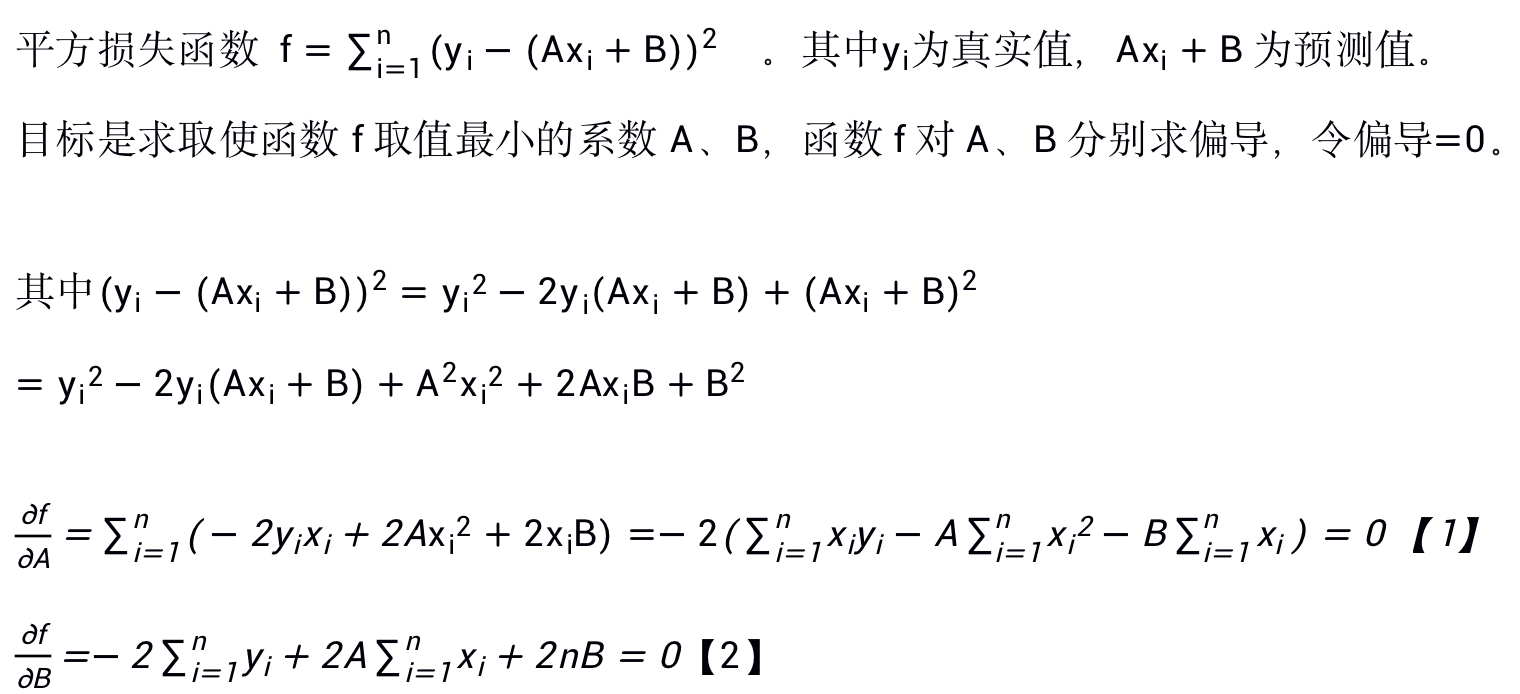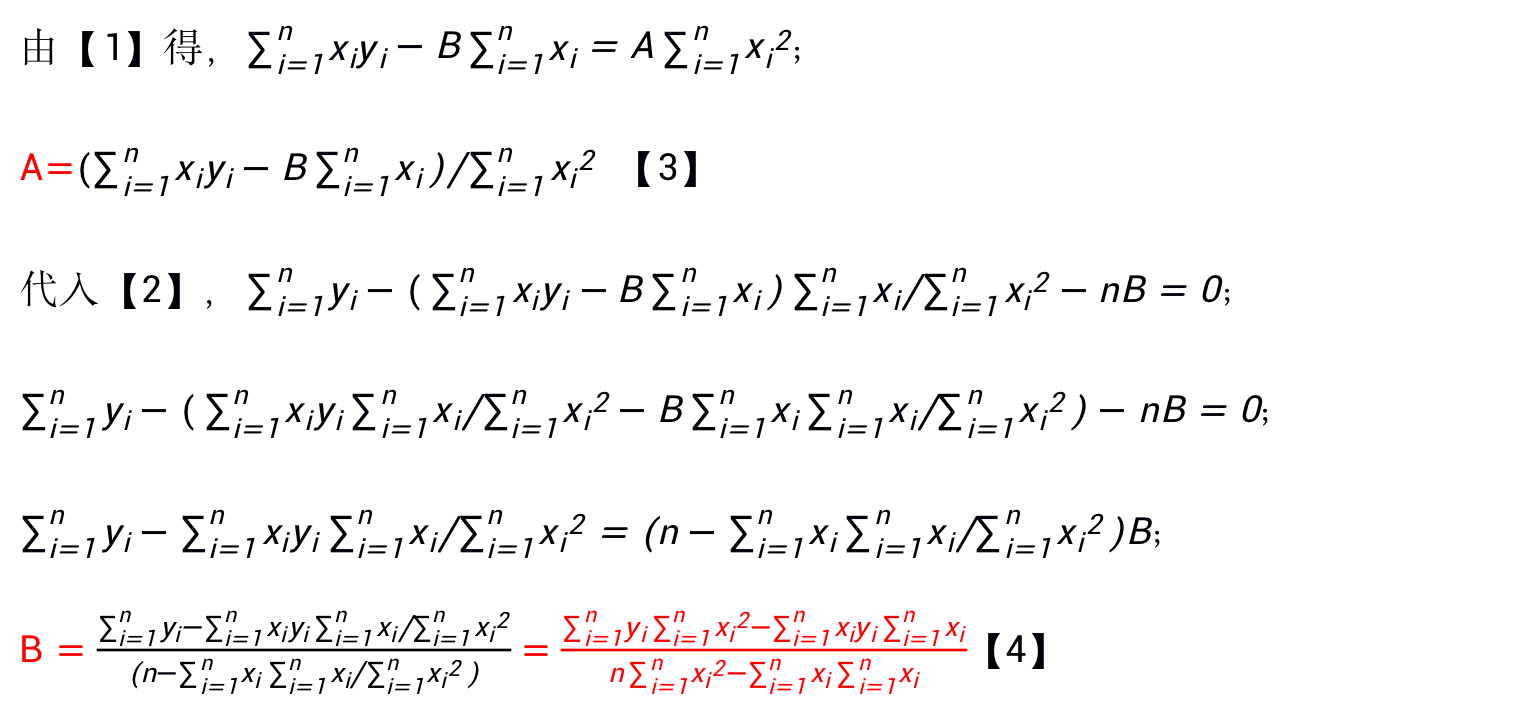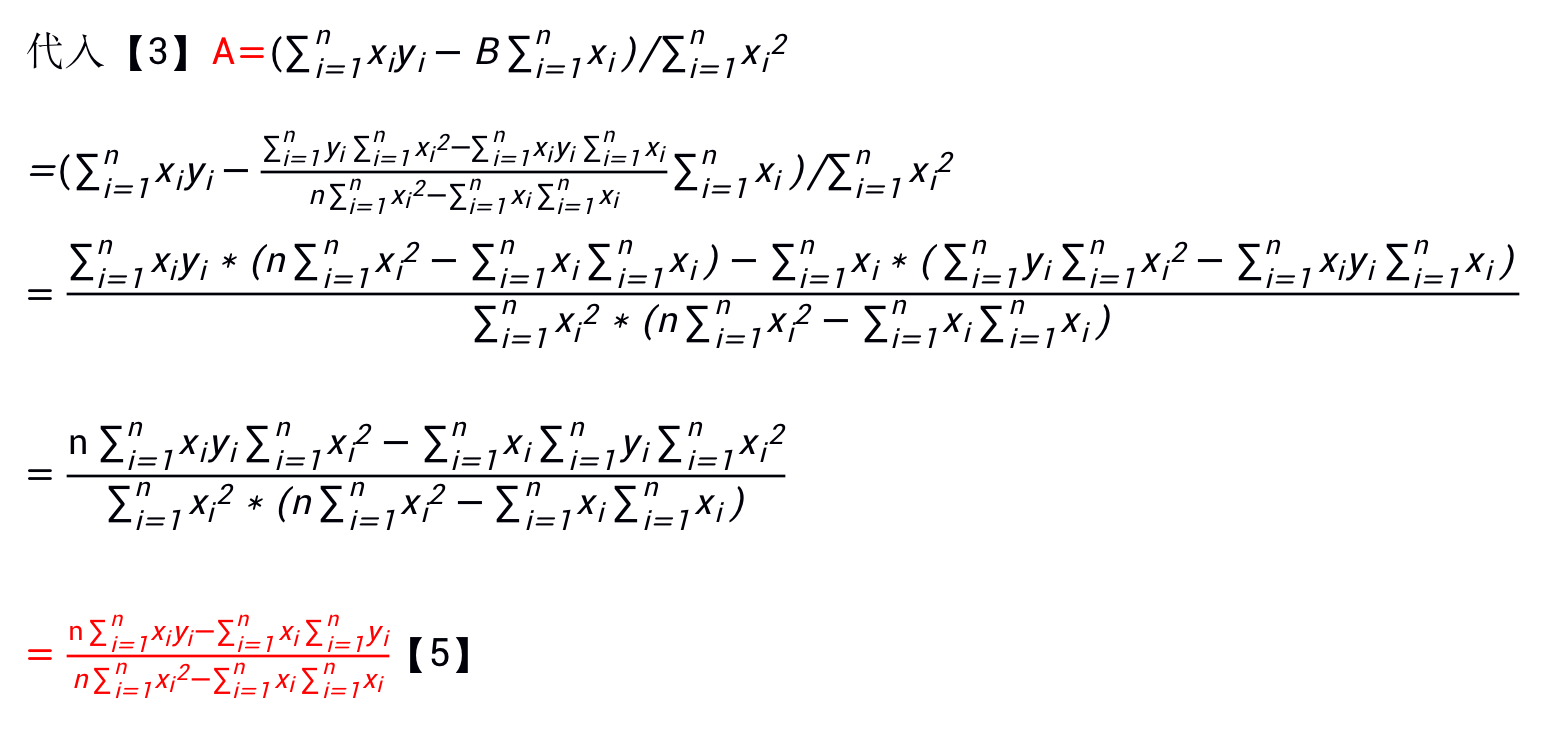此时对应python公式如下：

A = (n * sum(x*y) - sum(x)*sum(y)) / (n*sum(x * x)- sum(x)*sum(x))

B = (sum(x*x)*sum(y) - sum(x)*sum(x*y)) / (n*sum(x * x)- sum(x)*sum(x))

### 2)上述系数与常见形式转化

常见形式（来自：最小二乘法-维基百科 ）：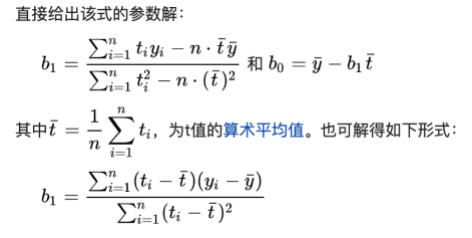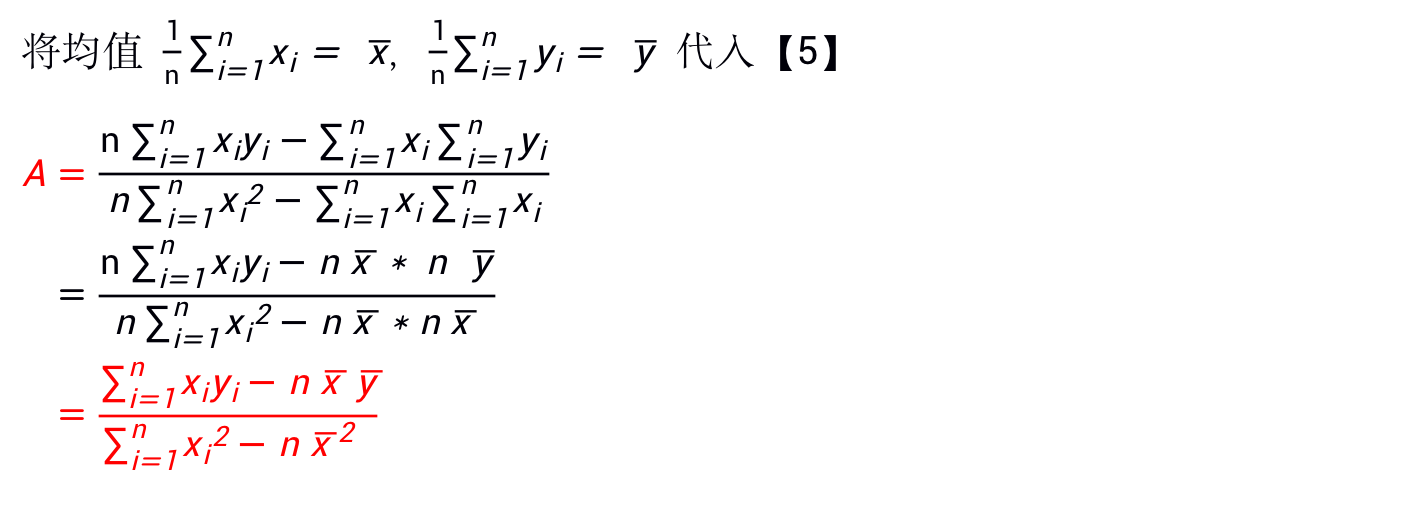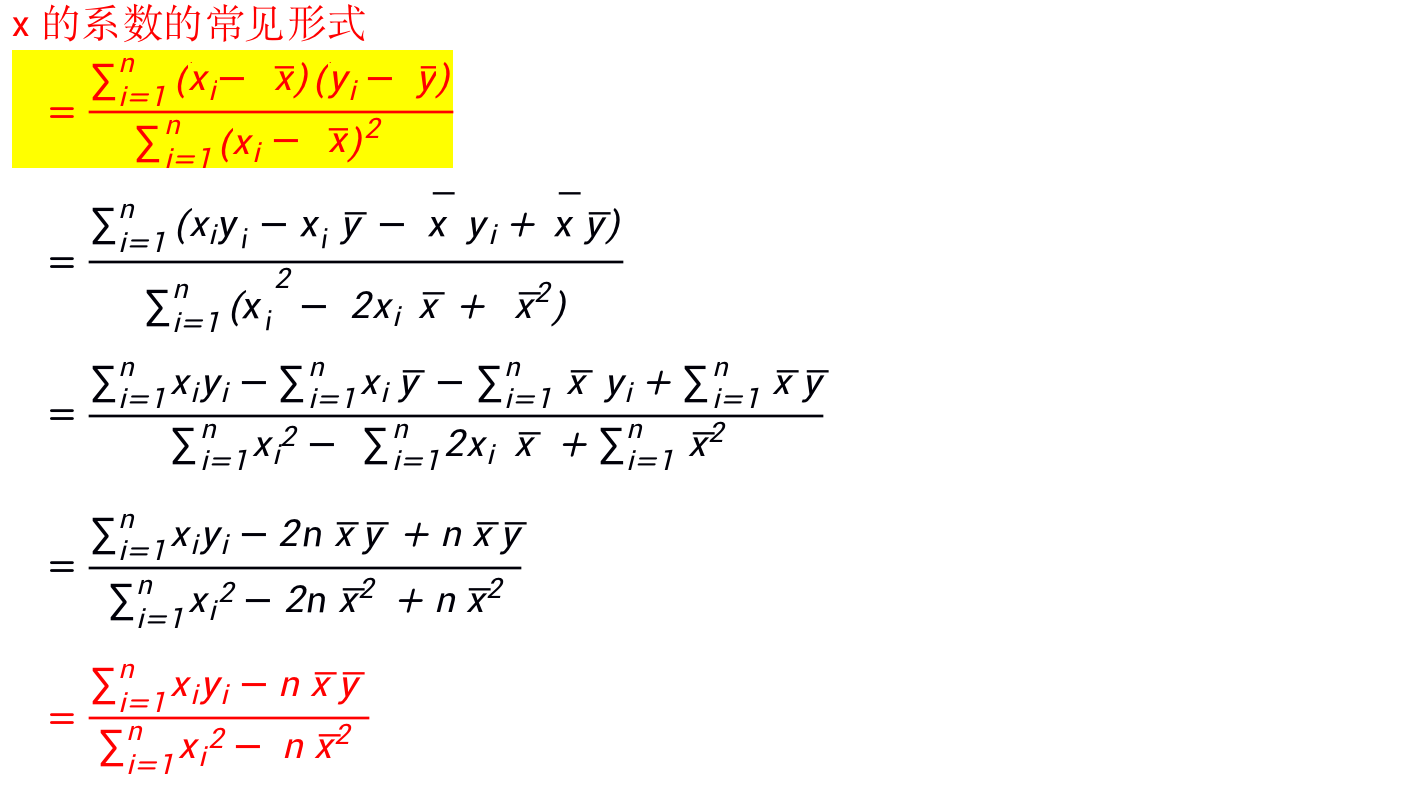此时对应python公式如下(常见形式)

    x = np.array(list(map(lnhandle, fields_avg)))

y = np.array(list(map(lnhandle, result_average)))

x_mean = np.mean(x)

y_mean = np.mean(y)

m1 = 0

m2 = 0

for xi, yi in zip(x, y):

m1 += (xi - x_mean) * (yi - y_mean)

m2 += (xi - x_mean) ** 2

b = m1 / m2

a = math.exp(y_mean - b * x_mean)

————————————————

版权声明：本文为CSDN博主「Backup and share」的原创文章，遵循CC 4.0 BY-SA版权协议，转载请附上原文出处链接及本声明。

原文链接：https://blog.csdn.net/ISIS7Protessional/article/details/115094852

展开全文python 线性回归 最小二乘法
• 引言 我们知道，自然对数的底 e 定义为以下极限值： 这个公式很适合于对幂函数的计算进行一些测试，得到的结果是 e 的近似值，不用担心当 n 很大时计算结果会溢出。 测试程序 下面就是 Tester.cs： 1 using System; ...

## 引言

我们知道，自然对数的底 e 定义为以下极限值：这个公式很适合于对幂函数的计算进行一些测试，得到的结果是 e 的近似值，不用担心当 n 很大时计算结果会溢出。

## 测试程序

下面就是 Tester.cs：

 1 using System;
2 using System.Numerics;
3 using System.Diagnostics;
4 using Skyiv.Extensions;
5
6 namespace Skyiv.Test
7 {
8   sealed class Tester
9   {
10     string Standard(long n)
11     { // n == 10^m
12       if (n > 100000) return "Skip";
13       var s = BigInteger.Pow(n + 1, (int)n).ToString();
14       s = s.Substring(0, Math.Min(31, s.Length));
15       return s + "." + s.Substring(1);
16     }
17
18     string Direct(long n)
19     {
20       if (n > 1000000000) return "Skip";
21       var y = 1m;
22       for (var x = 1 + 1m / n; n > 0; n--) y *= x;
23       return y.ToString();
24     }
25
26     string Binary(long n)
27     {
28       var y = 1m;
29       for (var x = 1 + 1m / n; n != 0; x *= x, n >>= 1)
30         if ((n & 1) != 0) y *= x;
31       return y.ToString();
32     }
33
34     string ExpLog(long n)
35     {
36       return (1 + 1m / n).Pow(n).ToString();
37     }
38
39     void Out(string name, Func<long, string> func, long n)
40     {
41       var timer = Stopwatch.StartNew();
42       var y = func(n);
43       timer.Stop();
44       Console.WriteLine("{0,-32} {1} {2}", y, timer.Elapsed, name);
45     }
46
47     void Run(int max)
48     {
49       for (var m = 0; m <= max; m++)
50       {
51         var n = (long)Math.Pow(10, m);
52         Console.WriteLine(string.Format("- {0:D2}:{1:N0} ", m, n).PadRight(58, '-'));
53         Out("Standard", Standard, n);
54         Out("Direct", Direct, n);
55         Out("Binary", Binary, n);
56         Out("ExpLog", ExpLog, n);
57       }
58     }
59
60     static void Main()
61     {
62       new Tester().Run(18);
63     }
64   }
65 }

这个程序使用四种方法来计算幂函数：

1. 第 10 至 16 行的 Standard 方法使用 BigInteger.Pow 方法来计算幂函数。这个计算结果（在有效数字范围内）是准确值，作为其他方法的标准。
2. 第 18 至 24 行的 Direct 方法直接将 x 乘上 n 遍来计算幂函数，是最没技术含量的暴力方法。时间复杂度是 O(N)。
3. 第 26 至 32 行的 Binary 方法将 n 视为二进制数，根据其为 1 的位来计算幂函数。这是经典的算法，时间复杂度是 O(logN)。FCL 的 BigInteger.Pow 方法也是使用这个算法。
4. 第 34 至 37 行的 ExpLog 方法使用 decimal 的扩展方法 Pow 来计算幂函数，是通过对数函数和指数函数来计算的：$an$。理论上说，时间复杂度是 O(1)。

## decimal 的扩展方法

下面就是 DecimalExtensions.cs：

 1 using System;
2
3 namespace Skyiv.Extensions
4 {
5   static class DecimalExtensions
6   {
7     static readonly int[] mask = { 1, 2, 4, 8, 16, 32, 64 };
8     static readonly decimal ln10 = 2.3025850929940456840179914547m;
9     static readonly decimal lnr = 0.2002433314278771112016301167m;
10     static readonly decimal expmax = 66.542129333754749704054283659m;
11     static readonly decimal[] exps =
12     {
13       2.71828182845904523536028747135m, // exp(1)
14       7.38905609893065022723042746058m, // exp(2)
15       54.5981500331442390781102612029m, // exp(4)
16       2980.95798704172827474359209945m, // exp(8)
17       8886110.52050787263676302374078m, // exp(16)
18       78962960182680.6951609780226351m, // exp(32)
19       6235149080811616882909238708.93m  // exp(64)
20     };
21
22     public static decimal Log10(this decimal x)
23     {
24       return Log(x) / ln10;
25     }
26
27     public static decimal Log(this decimal x)
28     {
29       if (x <= 0) throw new ArgumentException("Must be positive");
30       int k = 0, l = 0;
31       for (; x >= 1.10527199m; k++) x /= 10;
32       for (; x <= 0.1m; k--) x *= 10;        // ( 0.1000, 1.10527199 )
33       for (; x < 0.9047m; l--) x *= 1.2217m; // [ 0.9047, 1.10527199 )
34       return k * ln10 + l * lnr + Logarithm((x - 1) / (x + 1));
35     }
36
37     static decimal Logarithm(decimal y)
38     { // y in ( -0.05-, 0.05+ ), return ln((1+y)/(1-y))
39       decimal v = 1, y2 = y * y, t = y2, z = t / 3;
40       for (var i = 3; z != 0; z = (t *= y2) / (i += 2)) v += z;
41       return v * y * 2;
42     }
43
44     public static decimal Exp(this decimal x)
45     {
46       if (x > expmax) throw new OverflowException("overflow");
47       if (x < -66) return 0;
48       var n = (int)decimal.Round(x);
49       if (n > 66) n--;
50       decimal z = 1, y = Exponential(x - n);
51       for (int m = (n < 0) ? -n : n, i = 0; i < mask.Length; i++)
52         if ((m & mask[i]) != 0) z *= exps[i];
53       return (n < 0) ? (y / z) : (y * z);
54     }
55
56     static decimal Exponential(decimal q)
57     { // q (almost) in [ -0.5, 0.5 ]
58       decimal y = 1, t = q;
59       for (var i = 1; t != 0; t *= q / ++i) y += t;
60       return y;
61     }
62
63     public static decimal Pow(this decimal x, decimal y)
64     {
65       if (x == 0 && y > 0) return 0;
66       if (y == 0 && x != 0) return 1;
67       return Exp(y * Log(x));
68     }
69   }
70 }

这个程序的详细说明请见参考资料和。

## 编译和运行

在 Arch Linux 操作系统的 Mono 环境下编译和运行：

work$dmcs -r:System.Numerics.dll Tester.cs DecimalExtensions.cs work$ mono Tester.exe
- 00:1 ---------------------------------------------------
2.                               00:00:00.0085818 Standard
2                                00:00:00.0033230 Direct
2                                00:00:00.0002739 Binary
2.0000000000000000000000000005   00:00:00.0049157 ExpLog
- 01:10 --------------------------------------------------
2.5937424601                     00:00:00.0015421 Standard
2.5937424601000000000000000000   00:00:00.0000146 Direct
2.5937424601000000000000000000   00:00:00.0000092 Binary
2.5937424600999999999999999977   00:00:00.0000488 ExpLog
- 02:100 -------------------------------------------------
2.704813829421526093267194710807 00:00:00.0006872 Standard
2.7048138294215260932671947112   00:00:00.0000735 Direct
2.7048138294215260932671947103   00:00:00.0000234 Binary
2.7048138294215260932671947257   00:00:00.0000330 ExpLog
- 03:1,000 -----------------------------------------------
2.716923932235892457383088121947 00:00:00.0277308 Standard
2.7169239322358924573830881229   00:00:00.0007167 Direct
2.7169239322358924573830881218   00:00:00.0000159 Binary
2.7169239322358924573830883380   00:00:00.0000310 ExpLog
- 04:10,000 ----------------------------------------------
2.718145926825224864037664674913 00:00:03.3247007 Standard
2.7181459268252248640376646760   00:00:00.0068304 Direct
2.7181459268252248640376646665   00:00:00.0000191 Binary
2.7181459268252248640376679109   00:00:00.0000276 ExpLog
- 05:100,000 ---------------------------------------------
2.718268237174489668035064824426 00:07:56.2341075 Standard
2.7182682371744896680350648397   00:00:00.0686007 Direct
2.7182682371744896680350643783   00:00:00.0000222 Binary
2.7182682371744896680350286262   00:00:00.0000255 ExpLog
- 06:1,000,000 -------------------------------------------
Skip                             00:00:00.0000008 Standard
2.7182804693193768838197997202   00:00:00.6837104 Direct
2.7182804693193768838198166432   00:00:00.0000241 Binary
2.7182804693193768838199803836   00:00:00.0000213 ExpLog
- 07:10,000,000 ------------------------------------------
Skip                             00:00:00.0000009 Standard
2.7182816925449662711985502083   00:00:06.8334721 Direct
2.7182816925449662711985623547   00:00:00.0000289 Binary
2.7182816925449662712010419841   00:00:00.0000221 ExpLog
- 08:100,000,000 -----------------------------------------
Skip                             00:00:00.0000009 Standard
2.7182818148676362176529774118   00:01:08.3492423 Direct
2.7182818148676362176523859621   00:00:00.0000409 Binary
2.7182818148676362176710998015   00:00:00.0000230 ExpLog
- 09:1,000,000,000 ---------------------------------------
Skip                             00:00:00.0000007 Standard
2.7182818270999043223766453801   00:11:23.4187574 Direct
2.7182818270999043223770801045   00:00:00.0000442 Binary
2.7182818270999043220142064477   00:00:00.0000215 ExpLog
- 10:10,000,000,000 --------------------------------------
Skip                             00:00:00.0000007 Standard
Skip                             00:00:00.0000008 Direct
2.7182818283231311436196542093   00:00:00.0000349 Binary
2.7182818283231311439407330619   00:00:00.0000172 ExpLog
- 11:100,000,000,000 -------------------------------------
Skip                             00:00:00.0000008 Standard
Skip                             00:00:00.0000010 Direct
2.7182818284454538261539965115   00:00:00.0000398 Binary
2.7182818284454538262180262237   00:00:00.0000176 ExpLog
- 12:1,000,000,000,000 -----------------------------------
Skip                             00:00:00.0000010 Standard
Skip                             00:00:00.0000007 Direct
2.7182818284576860942863185484   00:00:00.0000403 Binary
2.7182818284576860944460582886   00:00:00.0000174 ExpLog
- 13:10,000,000,000,000 ----------------------------------
Skip                             00:00:00.0000009 Standard
Skip                             00:00:00.0000007 Direct
2.7182818284589093212295138270   00:00:00.0000436 Binary
2.7182818284589093212688645227   00:00:00.0000176 ExpLog
- 14:100,000,000,000,000 ---------------------------------
Skip                             00:00:00.0000009 Standard
Skip                             00:00:00.0000009 Direct
2.7182818284590316438350187680   00:00:00.0000480 Binary
2.7182818284590452353602874714   00:00:00.0000112 ExpLog
- 15:1,000,000,000,000,000 -------------------------------
Skip                             00:00:00.0000009 Standard
Skip                             00:00:00.0000009 Direct
2.7182818284590431765145511000   00:00:00.0000522 Binary
2.7182818284590452353602874714   00:00:00.0000114 ExpLog
- 16:10,000,000,000,000,000 ------------------------------
Skip                             00:00:00.0000009 Standard
Skip                             00:00:00.0000006 Direct
2.7182818284590335325626228124   00:00:00.0000547 Binary
2.7182818284590452353602874714   00:00:00.0000109 ExpLog
- 17:100,000,000,000,000,000 -----------------------------
Skip                             00:00:00.0000010 Standard
Skip                             00:00:00.0000006 Direct
2.7182818284590296936415060358   00:00:00.0000567 Binary
2.7182818284590452353602874714   00:00:00.0000108 ExpLog
- 18:1,000,000,000,000,000,000 ---------------------------
Skip                             00:00:00.0000010 Standard
Skip                             00:00:00.0000006 Direct
2.7182818284590434884535909399   00:00:00.0000615 Binary
2.7182818284590452353602874714   00:00:00.0000108 ExpLog
work\$ echo 'scale=30;e(1)' | bc -lq
2.718281828459045235360287471352


在上述结果中：

1. 最后一行是 e 的近似值，是使用 Linux 操作系统的高精度计算器 bc 计算的，请见参考资料。
2. 使用 BigInteger.Pow 计算出来的是准确值。在 05:100,000 这一组中，计算结果达 500,001 个十进制数字。当 n 达到 106 以后，由于计算量太大，已经无法在合理的时间内计算准确值了。
3. 使用 Direct 计算最慢（除 Standard 外，因为计算量不同）。当 n 达到 1010 以后，由于费时太多，已经不使用 Direct 方法计算了。
4. 使用 Binary 计算的速度非常快，其精度和 Direct 差不多。这两者答案不同说明 decimal 的乘法不满足结合律。
5. 使用 ExpLog 计算的速度理论上是最快的，实际的速度和 ExpLog 差不多，因为 n 还不够大。其精度在 n 不是很大时稍差。
6. 当 n 达到 1014 以后，ExpLog 计算出来的值在 29 个有效数字范围内已经等于 e 值，不再变化了。
7. 当 n 达到 1014 以后，由于舍入误差的累计，Binary 计算出来的值大约只有 14 个有效数字是可信的，再增大 n 值也不能更逼近 e 值了。也就是说，在逼近 e 值的意义上说，计算结果在有效数字范围内不再变化了。
8. 要计算的幂函数是增函数，请注意观察上述运行结果是如何体现这一点的。

## 参数资料

展开全文• 本文说明如何使用各种方法计算矩阵和指数。 正整数 如果 A 为方阵并且 p 为正整数，则 A^p 实际上是将 A 乘以其自身 p-1 次。例如： A = [1 1 1 1 2 3 1 3 6]; A^2 ans = 3×3 3 6 10 6 14 25 10 25 46 ...matlab
• “微分方程求解器” 此函数实现了微分方程求解的矩法L=微分算子的形式[导数的系数从6阶到常数的递减顺序] kcoeff= 已知多项式的形式 [x 的系数从 6 阶到常数的递减顺序] a=起始空间坐标值b=结束空间坐标值N=要取的...matlab
• ## MATLAB多项式函数及方程求根及绘图

千次阅读 多人点赞 2020-04-29 01:26:40
P = ploy(r)：以向量 r 内的元素为，返回多项式的系数 P = ploy(A)：返回矩阵 A 的特征多项式 如果紧接着 roots(P) 的话可以得到矩阵 A 的特征值，与 eig(A) 等价 poly2str函数：poly2str(p,自变量)：返回...
• 一、生成函数应用场景、 二、使用生成函数求解递推方程
• 请老师帮我看看，纠结到半夜还没出来，多谢啦~~~需要求解方程如下：9/16*belta^2*y^6-3/2*belta*(x^2-1)*y^4+(mu^2*x^2+(x^2-alpha)^2)*y^2=A^2需要求解 plot(w,a)的图像；用ezplot当然可以得到图像，但是对于...
• 第2章——2.4　函数方程2.4.1　方程函数的零点[学习目标]1.知道函数零点的定义，会求函数的零点.2.能说出函数零点的存在性定理，会判断函数零点的存在性及存在区间.3.能利用数形结合的方
• 能计算对数函数、指数函数和幂函数的模拟计算机在对数函数y=loga x(a>0,a≠1,x>0)中，自变量x和因变量y之间是对数关系，就是a的y次方的计算结果是x。现代模拟电路中，可以使用集成运算放大器的对数计算电路，...
• 一、使用生成函数求解不定方程解个数、 1、带限制条件、 2、带系数
• 能计算对数函数、指数函数和幂函数的模拟计算机 在对数函数y=loga x（a>0,a≠1,x>0）中，自变量x和因变量y之间是对数关系，就是a的y次方的计算结果是x。现代模拟电路中，可以使用集成运算放大器的对数计算电路...
• 使用python编写一元三次方求根函数，原理采用盛金求根法。形如#a*x**3 +b*x**2 + c*x +d = 0的式子，调用的时候shengjinSolution函数中a,b,c,d给定正确的值即可算出来。
•matlab 矩阵
• 总共8个实验，今天写的是方程求根里的通过牛顿迭代法求一元多次方程的根。若函数f(x)连续可导，将f(x)在点x_k 处进行一阶泰勒展开，有：令 f(x) = 0， 当f'(x_k) != 0 时，有：于是，我们可以得到迭代公式：关于牛顿...
• 指数函数:y=a^x.指数x是自变量 幂函数:y=x^a.幂是自变量 python中,计算指数函数:2**3 计算幂函数:math.exp(4) 转载于:https://www.cnblogs.com/hope100/p/4381746.html
• 假定S为符 号表达式,命令solve (S)求解表达式等于0的根,也 可以再输入一个......MATLAB 解方程的三个实例 1、 、 对于多项式 p(x)=x3-6x2-72x-27,求多项式 p(x)=0 的根,可用多项式求根函数 roots(p),其中 p 为多项式...
• 《MATLAB应用 求解非线性方程》由会员分享，可在线阅读，更多相关《MATLAB应用 求解非线性方程(16页珍藏版)》请在人人文库网上搜索。1、第7章 求解非线性方程7.1 多项式运算在MATLAB中的实现一、多项式的表达n次...
• 1、根据分部积分的规律，将幂函数x看成是u,将指数函数看成是 第一问，用分部积分法勉强可以通过，关键是要确定v和u.和第一类换元法。 2、还是用分部积分法来解：这部分困扰着自己好几天，一直得不到正确的结果，...
• 研究了一类涉及线性分式变换函数...对于其中一类简单的函数方程,给出了所有情形下的级数解。阐明了这类函数方程与一类涉及线性分式变换函数方程解之间的关系。通过不同情形下的具体例子,展示了求解函数方程的方法。
• print(pow(2, e, n)) print(pow(4, e, n)) print(pow(8, e, n)) ''' 128509160179202 518818742414340 358553002064450 ...由代码得出方程组： c1=2^e mod n c2=4^e mod n=2^2e mod n c3=8^e mod n=2^3e...算法 python 数学
• 其次,定义了模糊数和模糊数方程,基于结构元方法研究了模糊数运算和模糊数方程求解。同时,实现了一元二次模糊方程求解,利用区间[-1,1]上的单调函数将一元二次模糊方程求解问题转化为二元二次参数方程组...
• ## 指数方程怎么解

千次阅读 2020-05-09 14:09:19
指数方程怎么解 解指数方程的思路是,先把指数式去掉,化为代数方程去解. 这样,解指数方程就是这样把指数式转化的问题. 一共有三种题型,分述如下. 1、a^[f(x)]=b型. 化为对数式 则a^[f(x)]=b； 2、a^[f(x)]=a^...
• 使用生成函数求解不定方程解个数示例 , 扩展到正数解
• 在常微分方程求解器dsolve中使用了一些传统的技术例如laplace变换和积分因子法等,函数pdesolve则使用诸如特征法等经典方法求解偏微分方程. 此外, Maple还提供了可作摄动解的所有工具,例如Poincare-Lindstedt法和高...
• 是支持三角函数的！1个回答分类：综合2014-09-20问题解答：我来补答好了以后,按MODE你会发现有8个模式,其中EQN就是解方程.1.shift2.(Pol)3.14.shift5.(,)6.07.)8.=9.狂按分数线,直到按到顶不动为止(似乎是6个)10.按=...
• 给定了一组[x,y]，让我们去拟合一个指数函数，要如何做？ 不卖关子： 设待拟合函数为 y=k∗xay=k*x^{a}y=k∗xa 需要求出kkk和aaa. 思路是两边取对数，将指数函数拟合问题转换成线性函数 变换后： log(y)=log(k...算法...